# Normal Distribution Normal Distribution Curve A normal distribution

• Slides: 22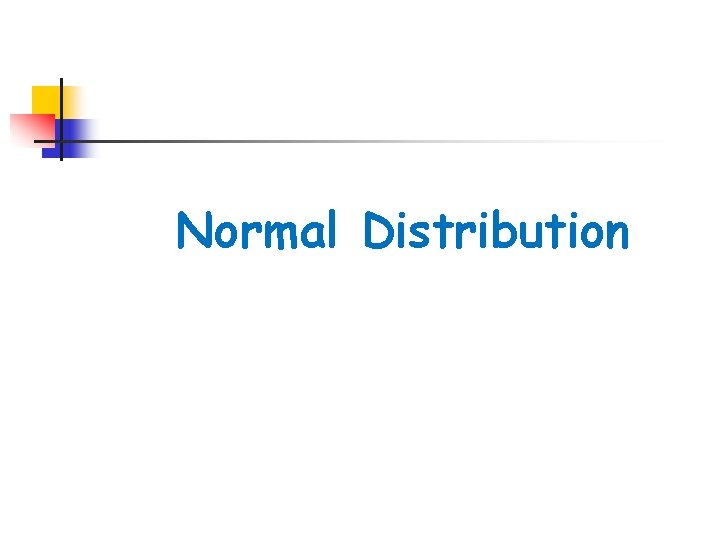Normal DistributionNormal Distribution Curve A normal distribution curve is symmetrical, bell-shaped curve defined by the mean and standard deviation of a data set. The normal curve is a probability distribution with a total area under the curve of 1.One standard deviation away from the mean ( ) in either direction on the horizontal axis accounts for around 68 percent of the data. Two standard deviations away from the mean accounts for roughly 95 percent of the data with three standard deviations representing about 99. 7 percent of the data.Standard Normal Distribution The mean of the data in a standard normal distribution is 0 and the standard deviation is 1. A standard normal distribution is the set of all z-scores.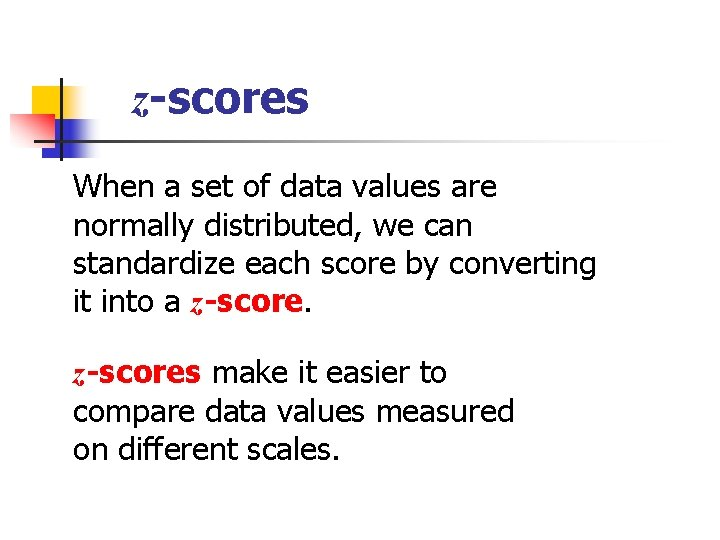z-scores When a set of data values are normally distributed, we can standardize each score by converting it into a z-scores make it easier to compare data values measured on different scales.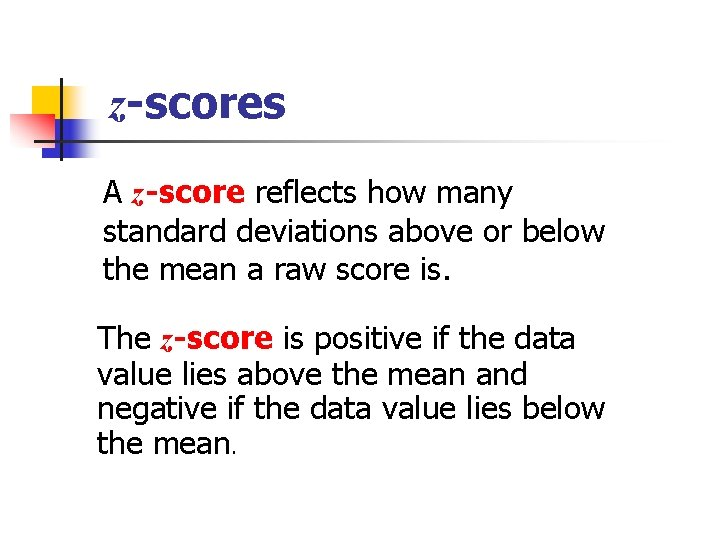z-scores A z-score reflects how many standard deviations above or below the mean a raw score is. The z-score is positive if the data value lies above the mean and negative if the data value lies below the mean.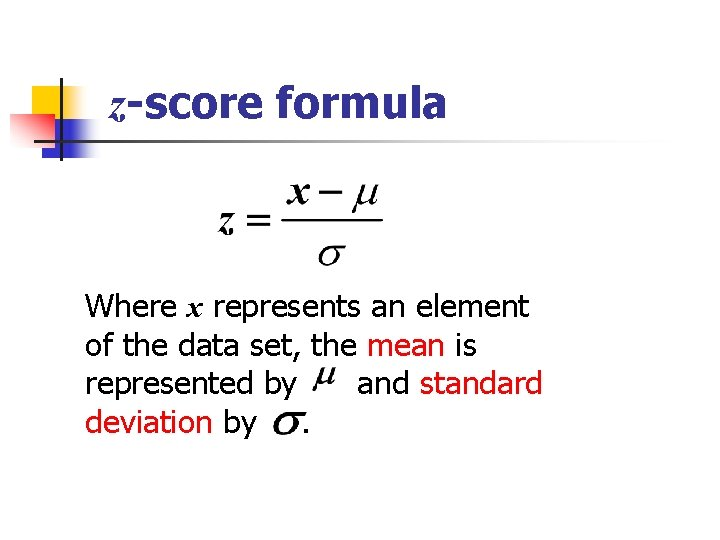z-score formula Where x represents an element of the data set, the mean is represented by and standard deviation by.Analyzing the data Suppose SAT scores among college students are normally distributed with a mean of 500 and a standard deviation of 100. If a student scores a 700, what would be her z-score? Answer Now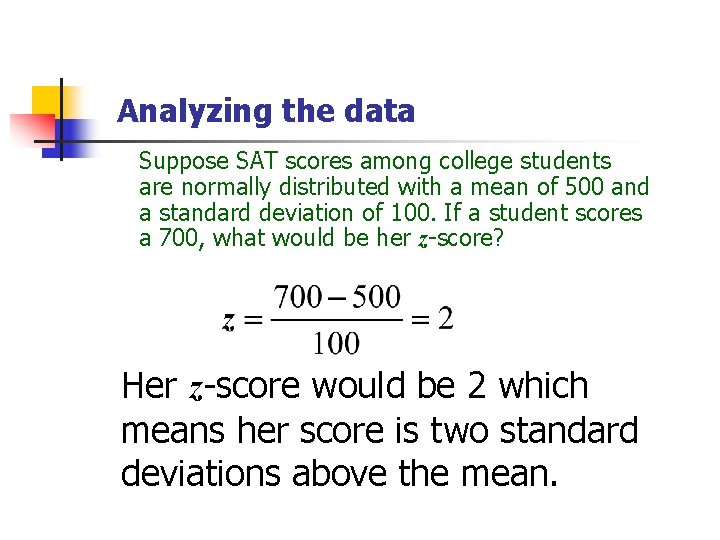Analyzing the data Suppose SAT scores among college students are normally distributed with a mean of 500 and a standard deviation of 100. If a student scores a 700, what would be her z-score? Her z-score would be 2 which means her score is two standard deviations above the mean.Analyzing the data • A set of math test scores has a mean of 70 and a standard deviation of 8. • A set of English test scores has a mean of 74 and a standard deviation of 16. For which test would a score of 78 have a higher standing? Answer NowAnalyzing the data A set of math test scores has a mean of 70 and a standard deviation of 8. A set of English test scores has a mean of 74 and a standard deviation of 16. For which test would a score of 78 have a higher standing? To solve: Find the z-score for each test. The math score would have the highest standing since it is 1 standard deviation above the mean while the English score is only. 25 standard deviation above the mean.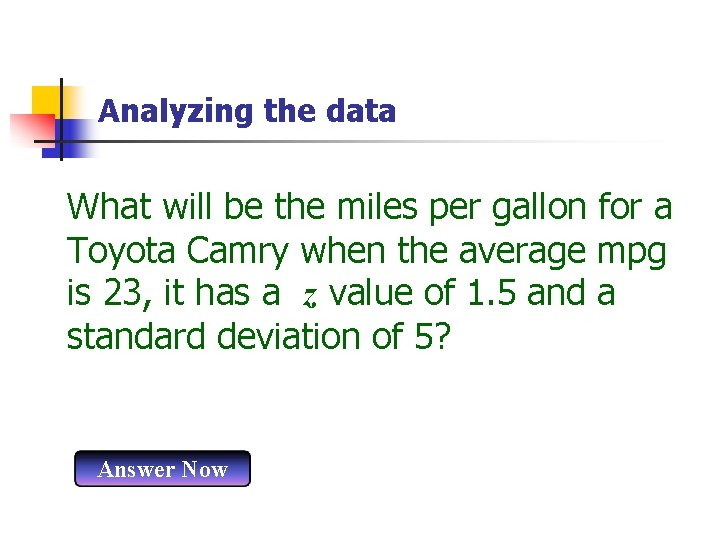Analyzing the data What will be the miles per gallon for a Toyota Camry when the average mpg is 23, it has a z value of 1. 5 and a standard deviation of 5? Answer Now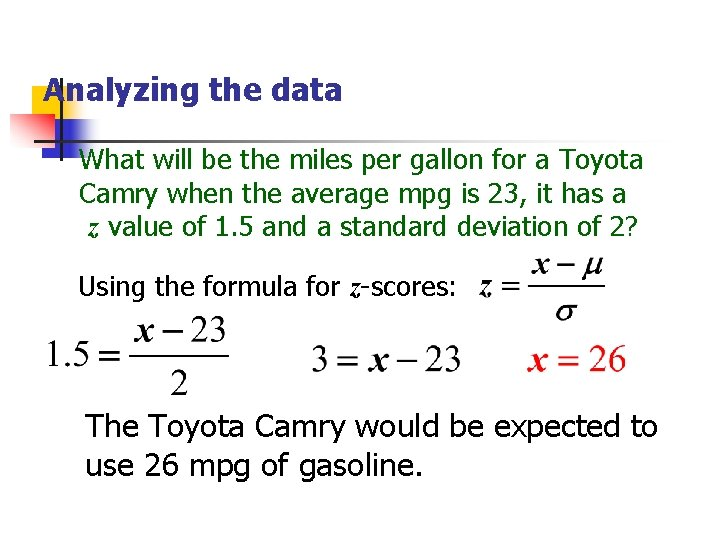Analyzing the data What will be the miles per gallon for a Toyota Camry when the average mpg is 23, it has a z value of 1. 5 and a standard deviation of 2? Using the formula for z-scores: The Toyota Camry would be expected to use 26 mpg of gasoline.Normal Distribution Probability With a graphing calculator, we can calculate the probability of normal distribution data falling between two specific values using the mean and standard deviation of the data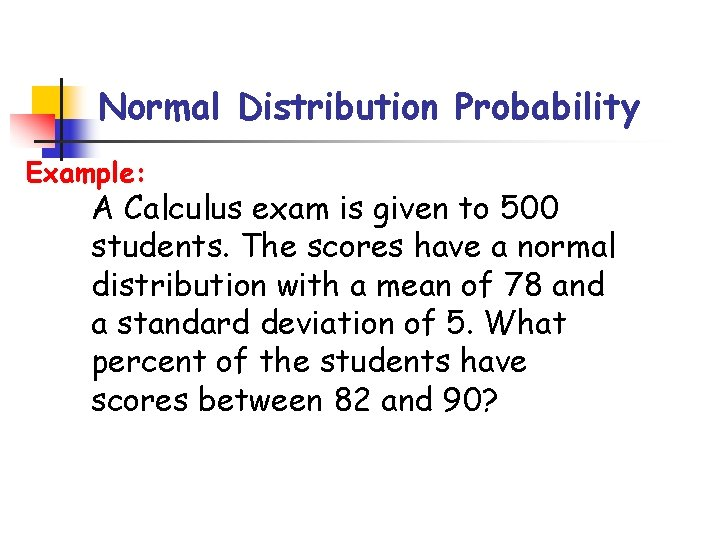Normal Distribution Probability Example: A Calculus exam is given to 500 students. The scores have a normal distribution with a mean of 78 and a standard deviation of 5. What percent of the students have scores between 82 and 90?TI 83/84 Normal Distribution Probability Example: A Calculus exam is given to 500 students. The scores have a normal distribution with a mean of 78 and a standard deviation of 5. What percent of the students have scores between 82 and 90? TI 83/84 directions: a. Press [2 nd][VARS](DISTR)  (normalcdf) b. Press  [, ]  [, ]  [, ]  [)][Enter] normalcdf(82, 90, 78, 5). 2036578048 There is a 20. 37% probability that a student scored between 82 and 90 on the Calculus exam.Normal Distribution Probability Extension: A Calculus exam is given to 500 students. The scores have a normal distribution with a mean of 78 and a standard deviation of 5. How many students have scores between 82 and 90? Using the probability previously found: 500 *. 2037 = 101. 85 There about 102 students who scored between 82 and 90 on the Calculus exam.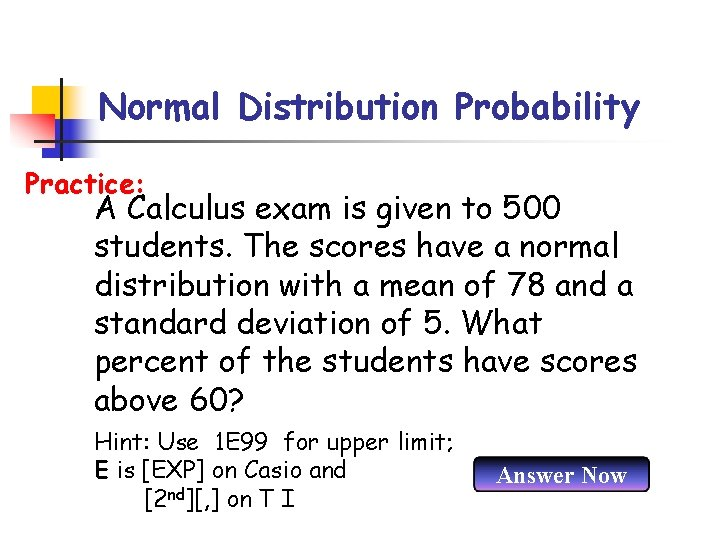Normal Distribution Probability Practice: A Calculus exam is given to 500 students. The scores have a normal distribution with a mean of 78 and a standard deviation of 5. What percent of the students have scores above 60? Hint: Use 1 E 99 for upper limit; E is [EXP] on Casio and [2 nd][, ] on T I Answer Now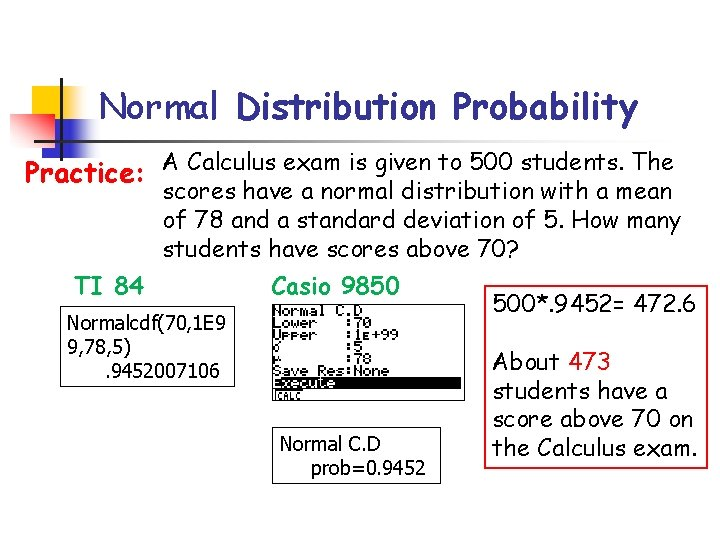Normal Distribution Probability Practice: A Calculus exam is given to 500 students. The scores have a normal distribution with a mean of 78 and a standard deviation of 5. How many students have scores above 70? TI 84 Casio 9850 Normalcdf(70, 1 E 9 9, 78, 5). 9452007106 Normal C. D prob=0. 9452 500*. 9452= 472. 6 About 473 students have a score above 70 on the Calculus exam.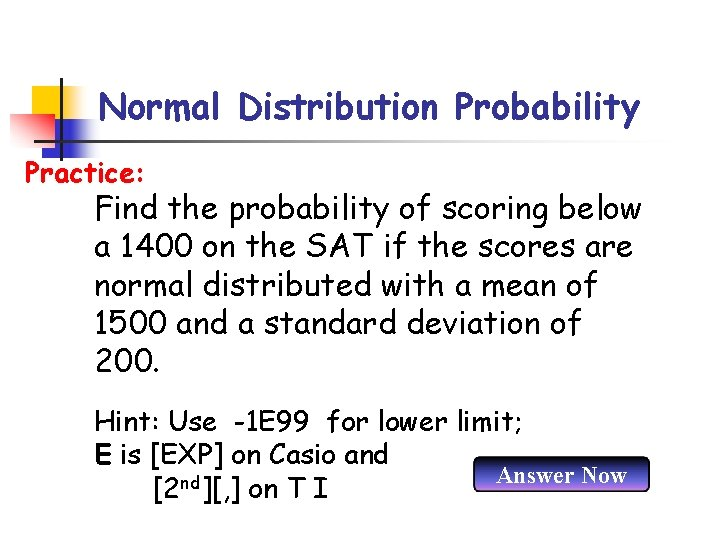Normal Distribution Probability Practice: Find the probability of scoring below a 1400 on the SAT if the scores are normal distributed with a mean of 1500 and a standard deviation of 200. Hint: Use -1 E 99 for lower limit; E is [EXP] on Casio and Answer Now nd [2 ][, ] on T I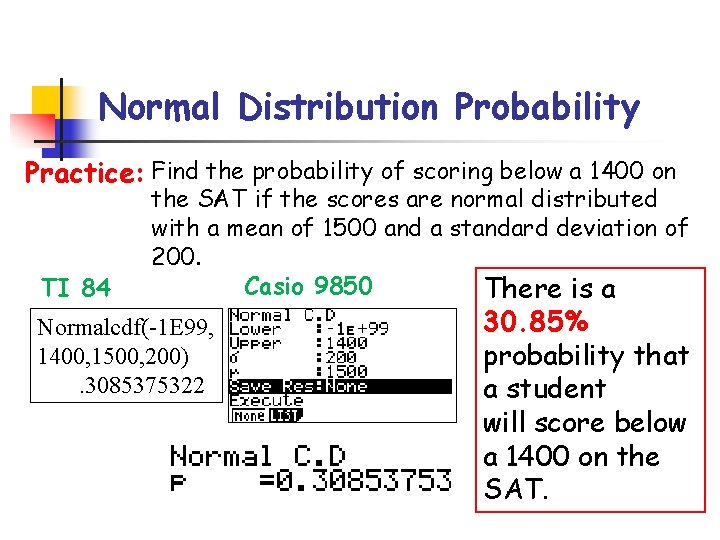Normal Distribution Probability Practice: Find the probability of scoring below a 1400 on TI 84 the SAT if the scores are normal distributed with a mean of 1500 and a standard deviation of 200. Casio 9850 There is a Normalcdf(-1 E 99, 1400, 1500, 200). 3085375322 30. 85% probability that a student will score below a 1400 on the SAT.